http://www.mathsisfun.com/positive-negative-integers.html

# How to Add and Subtract Positive and Negative Numbers

## Numbers Can be Positive or Negative: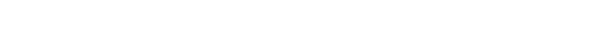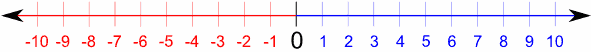Negative Numbers (−) Positive Numbers (+) (This is the Number Line, read about Using The Number Line)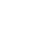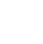“−” is the negative sign. “+” is the positive sign

## No Sign Means Positive

If a number has no sign it usually means that it is a positive number.

Example: 5 is really +5

### Example: 2 + 3 = 5

is really saying

“Positive 2 plus Positive 3 equals Positive 5”

You could write it as (+2) + (+3) = (+5)

### Subtracting Positive Numbers

Subtracting positive numbers is just simple subtraction.

### Example: 6 − 3 = 3

is really saying

“Positive 6 minus Positive 3 equals Positive 3”

You could write it as (+6) − (+3) = (+3)

## Balloons and WeightsThis basket has balloons and weights tied to it: The balloons pull up (positive) And the weights drag down (negative)

Here is what adding and subtracting positive numbers looks like:You can add balloons (you are adding positive value) the basket gets pulled upwards (positive)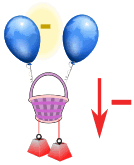You can take away balloons (you are subtracting positive value) the basket gets pulled downwards (negative)

Now let’s see what adding and subtracting negative numbers looks like:You can add weights (you are adding negative values) the basket gets pulled downwards (negative)And you can take away weights (you are subtracting negative values) the basket gets pulled upwards (positive)

That last one was interesting … subtracting a negative made the basket go up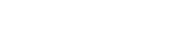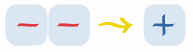Subtracting a Negative is the same as Adding

### Example: What is 6 − (−3) ?

6−(−3) = 6 + 3 = 9

### Example: What is 14 − (−4) ?

14−(−4) = 14 + 4 = 18

## And Positive and Negative Together …Subtracting a Positive or Adding a Negative is Subtraction

### Example: What is 6 − (+3) ?

6−(+3) = 6  3 = 3

### Example: What is 5 + (−7) ?

5+(−7) = 5  7 = −2

## The Rules:

It can all be put into two rules:

Rule Example +(+)Two like signs become a positive sign 3+(+2) = 3 + 2 = 5 6−(−3) = 6 + 3 = 9Two unlike signs become a negative sign 7+(−2) = 7 − 2 = 5 8−(+2) = 8 − 2 = 6

They are “like signs” when they are like each other (in other words: the same).

So, all you have to remember is:

Two like signs become a positive sign

Two unlike signs become a negative sign

### Example: What is 5+(−2) ?

+(−) are unlike signs (they are not the same), so they become a negative sign.

5+(−2) = 5  2 = 3

### Example: What is 25−(−4) ?

−(−) are like signs, so they become a positive sign.

25−(−4) = 25+4 = 29

### Example: What is −6+(+3) ?

+(+) are like signs, so they become a positive sign.

−6+(+3) = −6 + 3 = -3

Start at −6 on the number line, move forward 3, and you end up at −3